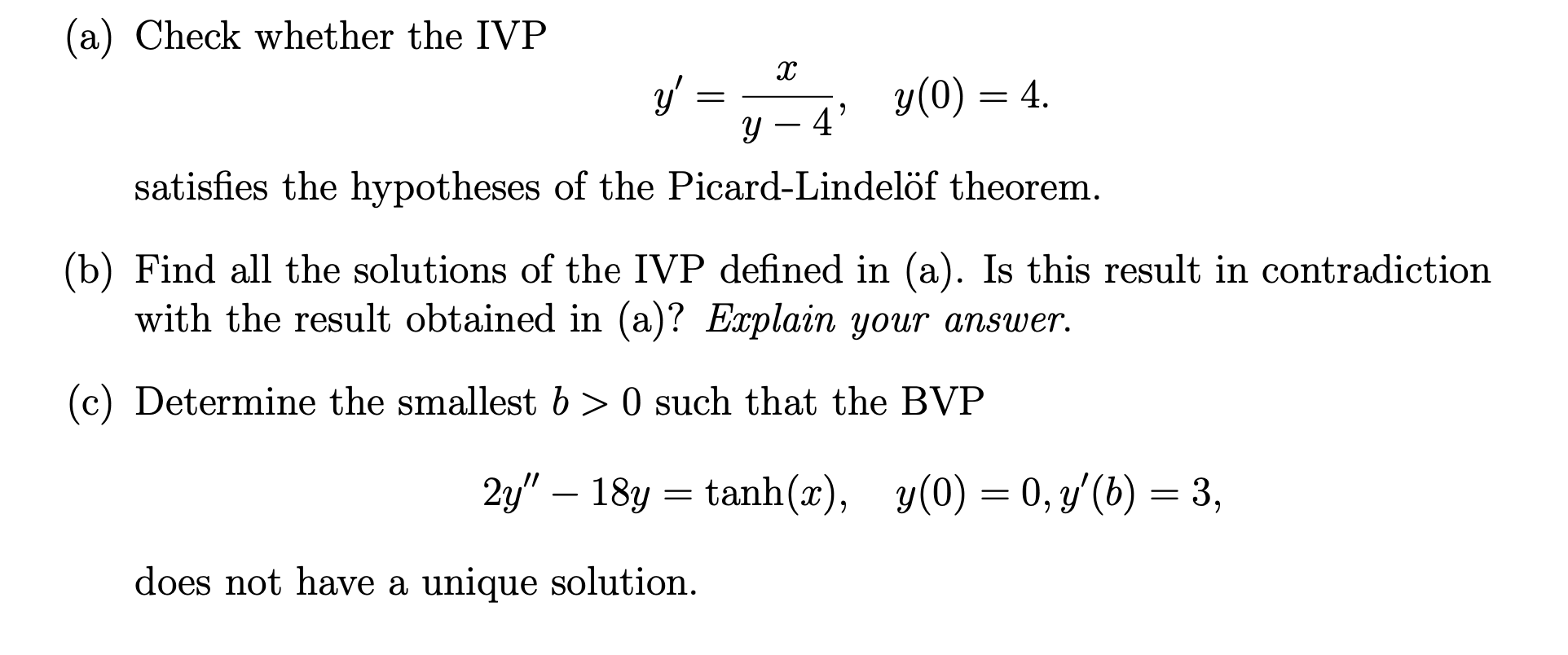# (Solved): (a) Check whether the IVP y=y4x,y(0)=4. satisfies the hypotheses of ...???????

(a) Check whether the IVP satisfies the hypotheses of the Picard-Lindelöf theorem. (b) Find all the solutions of the IVP defined in (a). Is this result in contradiction with the result obtained in (a)? Explain your answer. (c) Determine the smallest such that the BVP does not have a unique solution.

We have an Answer from Expert